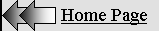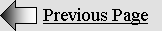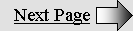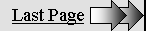THE  WAVE  THEORY

Explaining Matter and Relativity.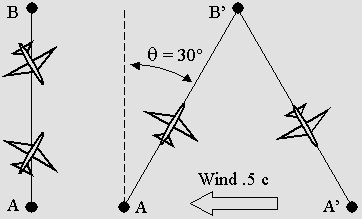Planes simulating waves traveling in a moving frame of reference.

Transverse AB'A' round trip absolute distance and time according to the gamma factor: 1 / (1 b 2 ) (1 / 2)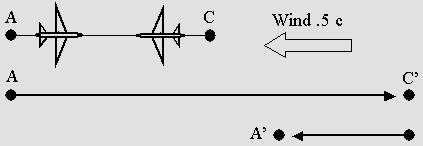Longitudinal AC'A' round trip absolute distance and time according to the gamma factor squared: 1 / (1 b 2 )

 A wave theory for matter and all forces. Aether exists. Matter is made of spherical standing waves. All forces work using waves. Because any wave exerts a radiation pressure, the result is motion. This motion is energy. Because this energy as motion surely involves the Doppler effect, one should understand what is going on while a standing wave structure moves through aether. This leads to the Lorentz transformations. This means that standing waves inside matter must obey the Lorentz transformations. So matter itself must contract on its displacement axis. It must also slow down its evolution speed. Surprisingly, this leads to Relativity. Lorentz's Relativity. The Postulates. Most of the wave theory postulates were listed in my first book La Théorie de l'Absolu ( The theory of Absolute) in may 2000. Here is the most recent version : The Wave Theory Postulates The electron is nothing but a spherical standing wave system. Its waves are submitted to the Doppler effect while it is moving. Such a moving electron will be shown a little later. Here, waves are concentric while the electron is at rest :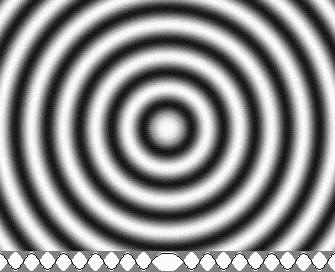The electron at rest.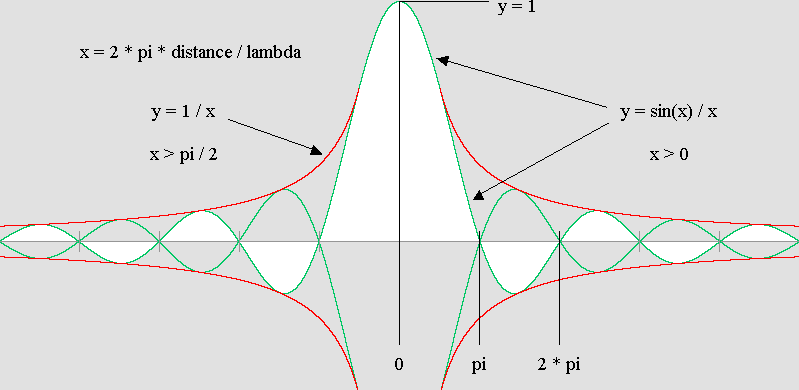The electron's amplitude diagram.

Lorentz was right.

The Lorentz transformations were found by both Hendrik Lorentz and Henri Poincaré, who were very good friends. They worked on Woldemar Voigt's transformations for almost ten years, using Maxwell's equations, and they finally found the correct value in 1904.

Einstein made a mistake using the Lorentz transformations in order to build up his version of Relativity. He did not admit that aether was a must. He spoke about "space contraction" and "time dilation". Definitely, this was a very weird and inane idea.

Lorentz spoke about aether, matter contraction and clocks slowing down instead. I strongly believe that he was right. By May 2000 I wrote a French book about "The theory of Absolute". I explained that the aether exists and that matter was made of standing waves. I also showed that Hendrik Lorentz explained Relativity far better than Albert Einstein did. However, nobody believed him because he could not explain why matter should transform this way.

Then I tried to understand what was wrong with Lorentz's formulas. I noticed that speed must be evaluated using space and time units. The point is that the Lorentz equations are already using space  x  and time  t  units, plus speed units and so space and time again. Clearly, any equation using such redundant values could not work properly. There should be something wrong about speed.

The speed of light.

Lorentz's equations actually induce a wrong interpretation of Relativity because the speed of light is just "c" for both frames of reference, while space and time magnitudes are not the same. There is no c' speed. It is not possible to know for sure whether the speed of light is to be converted into x' and t' measures instead of x and t. In addition, x' and t' stand for distances and time in the frame of reference which is stationary. Using x and t for the stationary one would be more logical.

Because of this uncertainty, it is not possible to prefer the frame of reference which is stationary any more. Both frames are apparently equivalent. This page will show that one must use a stationary Cartesian frame of reference instead in order to respect Lorentz's ideas.

However, the new set of equations will only show what will happen inside a moving system. Then it will be much more difficult to predict what a moving observer will see. As far as I know, nobody made this calculation correctly. Poincaré noticed that such an observer could not know whether he was moving or not, and he first spoke about Relativity in 1904.

But Lorentz was still sure about the aether. Fortunately, many people rediscover today this "new" Lorentzian Relativity.

Because matter is made of waves, one can today see that any motion should be the result of a series of longitudinal round trips or transverse zigzag displacements, whose speed is always that of the light.

Moreover Lorentz's equations indicate x values for space and t values for time. But they also indicate v and c values. Because any speed is the result of both x and t values, such values for speed appear to be redundant. One can simply remove them from Lorentz equations. And because it is not necessary to show a reciprocity, the new set of equations will be very simple:

 x ' = x cos q + t sin q t ' =  t sin q –  x sin q

Energy.

From a mechanical point of view, matter works using waves whose speed is constant. The radiation pressure is a force inducing motion, and motion is energy. This happens because of the Doppler effect. Compressed waves contain more energy, in a way very similar to a "subsonic boom".

More energy inside matter means new mass in accordance with Einstein's famous equation :

E = mc 2

It must be pointed out that Newton's equation (v is for velocity) for kinetic energy is :

E = mv 2 / 2

However, this equation only shows effective kinetic energy. For slow speeds, the total energy involved is doubled. One can easily understand that a billiard ball can push another one, but it is also slowed down during the process. This deceleration obviously needs as much energy as is needed for accelerating the other ball. So the total energy involved during the process is given by:

E = mv 2

On the other hand, Lorentz showed that the matter mass increases while it approaches the speed of light. The gamma factor indicates the gain value :

g  = 1 / (1 b 2 ) 1 / 2     with  b = v / c

For example 2 times more while b = .866 c, or 7 times more while b = .99 c.

The net mass gain  m'  is worth 7071 1 = 7070 times while b = .99999999 c. This additional mass is pure condensed kinetic energy. It represents almost all of its energy for speeds very near to c.

For such a high speed there is no need to spill half of Newton's kinetic energy any more, in order to stop a future 1 kilogram billiard ball when it is increased to 7071 kilograms. So the equation for kinetic energy as a mass gain  m'  surely is given by:

E = m'c 2

Let's prove it. The energy "E" must be measured in joules, the mass "m" in kilograms and the speed "v" or "c" in meters per second. Note that "c squared" means (1000 * 300000 km/s) ^ 2, about 9 * 10 ^ 16. Suppose a one kilogram meteorite is moving toward the Earth at .01 c (1860 miles or 3000 km per second). It contains .00005 kilogram as  m'  kinetic energy :

b = .01        m = 1

g = 1 / (1 b 2 ) 1 / 2  = 1.00005

m' = g m – m = g – 1 = .00005

E = m'c 2

E = .00005 * 9 * 10 16 joules

E = 4.5 * 10 12 joules.

This should produce a gigantic explosion. Now, according to Newton, the v squared speed is worth (.01 * 1000 * 300,000) ^ 2, about 9 * 10 ^ 12 and the mass  m  is not significantly increased (1.00005). It still is about one kilogram :

E = mv 2 / 2

E = 1 * 9 * 10 12 / 2

E = 4.5 * 10 12 joules.

This is consistent with Einstein's equation, at least while the mass increase is not significant. Otherwise the mc squared equation should prevail.

For example, one could suppose a .866 c speed. Then the gamma factor is 2. Moving at .866 c, a 2 kilogram meteorite can theoretically push a standing 1 kilogram one until it reaches .866 c. Note that the latter will then equal 2 kilograms and the first, only one, in accordance with the law of conservation of mass and energy.

So only 25% of kinetic energy instead of 50% will be spilled in order to stop the moving meteorite. Newton was not aware of this. His mv ^ 2 / 2 equation becomes gradually mv ^ 2 / 1 until it reaches almost the speed of light. Then his equation transforms to E = mc ^ 2 :

E = m v 2 / (1 + 1 / g)

Energy can be seen as kinetic or mass energy as well. There is no difference. Kinetic energy is simply the additional m' mass.

Kinetic means speed, and it turns out that Einstein's equation also shows that something inside matter always moves at the speed of light. This "something" must be waves. This equation shows that there is only one basic speed. The speed of light "c".

This new approach will lead us to a new set of equations for the Lorentz transformations, using wavelength instead of space, and wave phase instead of time.

Space, Motion and Speed.

This demonstration about Einstein's equation may look trivial. However, in my judgment, it is of the utmost importance. We know very well that matter behaves according to Einstein's equation. But the goal is to find out why matter behaves like this.

Motion was never correctly understood. Obviously Galileo was wrong. Minkowski's space-time concept is absurd. But Euclid's postulates and Descartes' aether and frame of reference system (at rest inside aether) still are true. One must now complete their space system and add motion to it.

Active and reactive masses.

Considering Einstein's equation, a new Wave Theory postulate should be that there is only one basic speed. The aether wave's speed. Clearly, there must be something inside matter which is always moving at this speed. We know that a particle cannot reach the speed of light. But the light can. This very strongly indicates a wave nature for matter. If so, it should also have a wave structure.

Waves need a medium. The Wave Theory postulates that aether exists. It also postulates that matter is made of spherical standing waves. One good reason for this is that such waves obey the Lorentz transformations.

Another reason is that the mass increase can be demonstrated using the Doppler effect. It works exactly the way a subsonic boom does. Such a phenomenon can easily be predicted using the Doppler effect formulas : 1 b and 1 + b. Here is a diagram showing it in a spectacular way: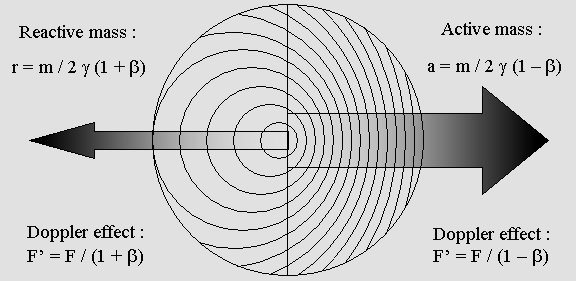Action and reaction. Suppose a one kilogram billiard ball at rest. While moving at .866 c speed, the beta  b  value is worth .866 and the gamma g factor is 2. This means that the ball will be increased to 2 kilograms. A standing wave system at rest is radiating half of its energy forward (active mass a on the positive side) and the other half backward (reactive mass r on the negative side) on a given  x  axis. According to Lorentz, for such a high speed, one must first consider that clocks will run two times slower. The system frequency will also be two times lower, and because energy is proportional to frequency, it will be two times less. Here, 2 is for both halves, and gamma is for energy loss :  a = m / 2 g               r = m / 2 g Moreover the Doppler effect is 1 / (1 – b) forward and 1 / (1 + b) backward : a = m / 2 g (1 – b)               r = m / 2 g (1 + b) a = 1 / 2 * 2 (1 – .866)               r = 1 / 2 * 2 (1 + .866) Active mass  a = 1.866 kg               Reactive mass  r = .134 kg a + r = 2 kg g m = 2 kg g m = a + r So the mass gain clearly involves the Doppler effect. Please note here that Mr. Milo Wolff also found that the mass increases according to the Doppler effect, which also causes de Broglie's wave. This mass increase can be predicted using the Lorentz transformations. No surprise there : the Lorentz transformations actually are the result of the Doppler effect. But it can also be predicted using the Doppler effect and a new motion postulate founded on the fact that the time needed for any wave round trip between two points, hence its cycle, will be two times longer inside such a moving wave structure. Here is how a moving electron behaves: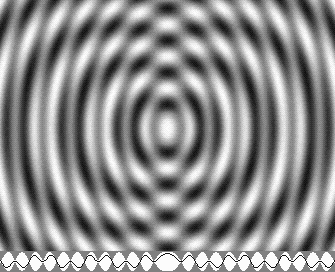The electron mass/energy increases in accordance with the Doppler effect.

 A new law of action and reaction. From a mechanical point of view, those active and reactive masses generate active and reactive forces. They clearly indicate that Newton's famous third law is wrong :  " Any action has an equal and opposite reaction ". Because of the mass increase, the inequality has been demonstrated for a long time. Moreover, the Doppler effect is sinusoidal : l' = l (1 – b cos j), and oblique forces also are. So any force should also follow this formula, using a "phi" angle which is 0° forward and reaches 180° backward :  F' = F / (1 – b cos j)  Nobody seems to have proposed the correct law. In my opinion it should be worded like this : " Any action has a simultaneous and opposite reaction in accordance with the Doppler effect ".   The shade effect and the radiation pressure. The page on wave mechanics shows that progressive waves must lose some of their energy while crossing standing waves. Because of the lens effect, a significant quantity of energy is transferred from aether waves to electrons. Then this energy as spherical wavelets is radiated by electrons. Because this amplification process weakens aether waves coming from any matter object, the waves become stronger in the opposite direction. This is the shade effect. On the other hand, electrons radiate spherical wavelets containing exactly the same quantity of energy. Any aether wave exerts a radiation pressure. It can push all electrons inside matter. This pressure should compensate for the shade effect, but a very small difference can explain gravity. When two electrons are put close together, their waves are melting together, and this produces fields such as electrostatic or gluonic. Such a system radiates most of its energy along the axis. The result is a repulsive effect along the axis and an attractive effect in any other direction. The effect will be inverted using an electron and a positron. Let us make it clear : An attraction effect occurs when the shade effect is stronger then the radiation pressure. A repulsion effect occurs when the radiation pressure is stronger then the shade effect. Gluonic fields. Electrons are very close together inside a quark and a proton. Inside such systems, waves from each electron and positron are strongly adding themselves. This produces "gluons" or gluonic fields. They are plane cylindrical standing wave systems which are also amplified by aether waves making their energy much more intense than that of one electron alone. It is a well known fact that collisions between positrons and electrons produce quarks. There is no evidence that they actually annihilate. So electrons and positrons could be indestructible. In such a case, energy released by radioactive elements or by hydrogen fusion could originate only from gluonic fields. Matter at rest contains energy, but it is not likely to be useable.   Huygens' principle. Any wave theory should first acknowledge that wave phenomena are composite and behave in accordance with Huygens' principle. That is, they can be predicted using thousands of "wavelets" adding or substracting themselves. Huygens only spoke about wavelets originating from a wave front. This principle supposes that the number of such wavelets is infinite. However, Augustin Fresnel noticed that many sources would also produce similar effects even while they are not on a wave front. For example, one can show that thousands of spherical sources on a flat laser window will produce a light beam and also an Airy disk at a certain distance. But the diffraction pattern would be quite different (here L is for distance and D for diameter) for distances less than :  L = D 2 / 2.44 l Those wavelets are sinusoidal in nature, and so sine and cosine functions must be used. As far as we know, the Huygens' principle has always proved to be true. So any other equation which does not predict the same result is simply wrong. In other words, one should play safe and always use Huygens' principle. Many animations and diagrams showed on this web site were computed this way. For example examine this astonishing animated Airy disk longitudinal view. Computers today can process thousands of wavelets in a fraction of a second. Huygens and Fresnel could not. Moreover, computers reveal that spherical wavelets emitted by electrons can explain gravity because their number is not infinite. There is a distance between each of them, and waves are curved, not flat. Differential calculus can show what would happen with an infinite number, but the computer shows that the result is slightly different using more or less wavelets. It can also reveal the general trend towards infinity. Huygens principle explains gravity. There are billions of electrons but their number still is not infinite. Because matter mechanics involves huge quantities of energy, this slight difference can explain gravity. Indeed, gravity is a very weak force, almost unperceivable between rather small objects. This means that gravity is definitely not the "fundamental force of the Universe". It does not involve specific waves or particles. There is no need to call upon a whole "General Relativity theory" for that, albeit tremendous pressures inside massive stars can certainly cause space and time effects. Motion is the result of composite wave displacements. Any displacement always occurs at the speed of light, and the result is motion. Motion is essentially composite in nature. It cannot be faster then the speed of light. It must be a series of back and forth waves round trips or a sequence of sideways zigzag displacements always taking place at the speed of light "c". The following argument has been developed hundreds of times since Lorentz. However, it must be repeated here because Albert Einstein's theory still prevails today. Planes flying through the wind. Showing a moving wave on this page appears to be quite a difficult enterprise. Fortunately, waves can be represented by planes flying through the wind, in a much more intuitive manner. Suppose that the planes constant speed is 100 miles per hour and that the wind is blowing at 50 mph. This means b = .5 and the q angle (arc sin b = 30°) will be very noticeable when they are flying across the wind for an observer on the ground. However, as seen from a Montgolfier Balloon, whose speed is the same as that of the wind, the scene would be quite different. There will be no angle any more, and the planes speed will still be 100 mph. This is the "absolute" point of view. The following diagram shows that when there is no wind at all (or no aether wind) any round trip between A and B or between A and C (while AB = AC) is the shortest and the fastest. A race between two such planes will give a null result. Michelson's interferometer works exactly this way. It is a wave race instead of a plane race :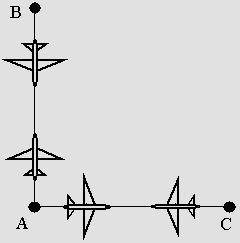No wind.

 The wind is blowing sideways. However, this is no longer true at high speed (high motion would be the correct word). Let's repeat that here we suppose a beta  b = .5 c motion. The theta  q  angle is worth : arc sin .5 or 30°. The ABA round trip on a transverse axis becomes a zigzag AB'A' displacement. The absolute length of such a displacement is worth 1 / cos 30° or 1.1547 times the ABA original one :As seen from a Montgolfier Balloon, the planes are not tilted.

 Note that when they are seen from the ground, the planes must be tilted according the q = 30° angle. They seem to maintain their original AB round trip. But actually their trip as compared to air is AB'A' and the additional distance (1 / cos q = 1.1547 instead of 1) makes it so that the time needed to perform the round trip is also 1.1547 times the original time. The round trip along the wind axis. The round trip distance along the displacement axis is even more complex because the motion direction must be inverted for a shorter time and distance. Such a motion follows the Doppler effect forward and backward : Distance and time forward :  1 / (1 – b) = 2       Speed : 1 – b = .5 Distance and time backward :  1 / (1 + b) = 2 / 3       Speed : 1 + b = 1.5 This means that while the plane is flying forward the actual distance AC' will be doubled. While returning, the actual distance will be reduced to 2 / 3.Along the wind axis.

 The absolute AC'A' round trip distance on the wind axis will be (2 + 2/3) / 2 = 1.3333 while it was 1.1547 across the wind. It turns out that a longitudinal round trip is longer than the equivalent transverse one. This is consistent with Albert A. Michelson's calculations for his interferometer: Transverse distance and time : 1 / cos q = 1.1547 Longitudinal distance and time : 1 / (cos q) 2 = 1.3333 This is also consistent with Lorentz's equations. One must remember that Lorentz was trying to find out why this interferometer did not reveal the aether wind. Lorentz explained that it encountered a length contraction, whose value was : cos q = .866. The  x  distance would be reduced to 1.3333 * .866 = 1.1547, making the absolute round trip distance exactly the same on all axes. Lorentz believed that matter really contracts at high speed. Nobody believed him because he could not explain why. However, we know today that matter involves waves. We also know that standing waves do transform in accordance with Lorentz's equations. Now we know why matter should behave like it does. Clocks will slow down. So any clock should contract. No problem there : standing waves do contract this way, and the clocks are made of standing waves. Then their pendulum absolute round trip distance would be the same on all x, y and z axes, but the time needed to perform a two-way cycle would still be 1.1547 longer on all axes.  Planes are only a comparison. Waves would behave like this too. Clearly, any periodic phenomenon or wave frequency will slow down. Matter as waves must evolve slower at high speed. From a mechanical point of view, a pendulum must slow down while accelerating, because the absolute distance is increased. Galileo's transformation was wrong because it did not predict such a time effect. It did not predict a contraction either. On the contrary a Cartesian frame of reference still works, but it must be at rest inside aether. The matter wave properties are well acknowledged today. The Lorentz transformations should appear merely obvious, as Euclid's geometry is. No tricky reasoning. No breathtaking equations. Pure mechanics. Pure logic. Lorentz and the radar. Radar pulses behave exactly in the same way as planes. Their absolute speed through aether is constant, but their relative speed is no longer the same for any moving observer. Here, we suppose 86.6% of the light speed. Then the Lorentz contraction is worth 50% according to the gamma factor (1 / g). The following animation clearly shows that four radar pulses and their echoes must come back exactly in the same time :   The 5 observers will be unable to detect the contraction. The time shift will cancel the signal delay forward and backward. Their clocks will run slower while the signal speed will also travel two times slower.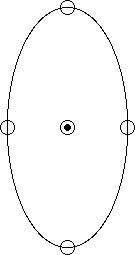A radar signal inside a moving system at .866 c.

 The Doppler effect. Any wave theory should include the Doppler effect. This phenomenon explains almost all of matter's behavior.  Although it is well known, very few people can use it correctly in order to explain the Lorentz transformations and Relativity :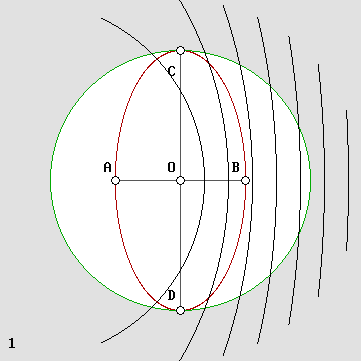The Doppler effect :  l' = l (1 b cos j)

Forward : 1 b      Backward : 1 + b

A little exercise.

Suppose that you are traveling at .866 c speed inside a space ship among those A,B,C,D and O observers.

The goal is to show that it will be impossible to detect any difference. All observers will think that they are at rest. Apparently, no contraction. No clocks slowing down. This leads to Relativity.

Actually, there is a contraction. Clocks do slow down.

Standing waves behave in accordance with  Lorentz.

I show in my page on standing waves that such waves behave exactly this way. As far as I know, this was Mr. Yuri Ivanov's discovery. As Mr. Ivanov pointed out, matter must behave this way too because waves are responsible for atomic and molecular binding. One will also find this in Mr. Serge Cabala's web site.

Lorentz was working on Voigt's transformations, using Maxwell's equations. He was trying to find out why Michelson's interferometer did not reveal the aether wind. As FitzGerald did, he found that matter should contract on the displacement axis. He also found that it should reduce its evolution speed.

Clearly, Lorentz was right. Unfortunately, nobody believed him because he could not explain why this should happen. He was not aware of the matter's wave properties. And he put down his transformations the wrong way because he was greatly influenced by his friend Henri Poincaré.

A Cartesian frame of reference.

Galileo's Relativity Principle is wrong. It cannot work because aether exists. One simply cannot transfer data from a frame at rest to a moving frame. The frame should stay at rest.

In order to understand what a moving observer will see, one must then make more complex calculations involving the Lorentz transformations and the Doppler effect. One will then realize that this observer does not see what is really going on. Illusions are not the truth.

Galileo's Relativity Principle does not hold true because of the presence of the aether, which was discovered by Descartes. There is no moving frame of reference any more. It must be immobile. Because of the Doppler effect, and also because any observer is transformed at high speed, any phenomenon which is not seen from an absolute point of view cannot be observed as true. A distortion occurs. The illusion is so perfect that this observer can always presume that he is at rest. But he is not.

Descartes himself knew that aether should exist as a medium for light waves. He actually discovered aether. His x, y and z system of coordinates definitely suppose a frame of reference at rest inside aether. There is no need to transpose values from one frame to another any more, as Lorentz did. The frame at rest must always prevail. Quite simply, a moving observer is always wrong.

The Lorentz transformations revisited.

So the Lorentz equations should simply be seen from a geometrical absolute point of view, using the  q  angle. Suppose that the aether wind is blowing at .866 c. This is the beta value, and the theta angle is given by :

b = .866        q = arc sin b         q = 60°

One will also need a clock synchronization convention for the frame at rest :

t = 0

There is no absolute time shift, so  t = 0  everywhere. The goal is to obtain  t' = 0  while  x = x' = 0 as a synchronization convention. The zero distance there facilitates such a convention.

Then, using  x  units in absolute light seconds (x = 1 is 186,000 miles or 300 000 km) and  t  units in absolute true seconds, one can finally rewrite the Lorentz transformations this way :

 x' = x cos q t' = –x sin q q = arc sin (v / c)

The Lorentz transformations revisited. Isn't this simple?

But this is true only when  t = 0.

x' indicates the contraction but t' indicates only the time shift.

Those equations are much simpler than Lorentz's. The main difference is that values are absolute and referenced to the same frame, which is at rest.

Please pay attention to this: t' time is absolute because it is truly displayed by moving clocks, but it is nevertheless slower than t, which is the only absolute true time.

Those transformations are effective. They show the difference between any wave system dimensions and wave state while it is at rest and while it is moving. It should be emphasized that the true variable x concerns the electron's wavelength at rest; the true variable t concerns its phase at rest. There is no space/time effect. But one can suppose a matter entity (which is made of electrons) undergoing those changes as well; then the x and x' variables apply to distances between objects measured in light seconds, and t is measured in absolute seconds. Let's repeat because this was seldom understood in the past: despite the fact that it is absolute (it represents the time truly displayed by moving clocks), the t' time is two times slower here.

1. The first equation indicates a contraction according to the cosine of 60°.

2. There is no change on any transverse axis: y' = y; z' = z.

3. The time equation indicates a time shift according to the sine of 60°.

4. A consequence of this is that clocks will run slower according to the cosine of 60°.

The x values are for a point while it is at rest. The x' and t' absolute values are for a point while it is moving. Note that in such a moving world events are not simultaneous. This does not only apply to clocks. From a mechanical point of view, this is necessary in order to cancel the waves' different speed forward and backward. Let's make it clear, this is not really a time effect, but rather a cause and effect distortion. Surprisingly, causes take place sooner at the rear of any moving system.

When x is 1 light seconds, a contracted .866 c moving clock actually is at x' = x cos 60° = .5 light second, and it runs two times slower. It does not indicate the absolute t time any more. But this clock really indicates .866 relative slower seconds less than another one which is at x = x' = 0. This means that the time equation can be even more simple:

Relative time actually recorded by the .866 c moving clock for t = 0, x = 1 and x' = .5:

t' = b

t' is not in absolute t seconds, but the clock's needle really indicates t' = –.866 second.

Poincaré found this value while trying to synchronizing clocks by exchanging light signals back and forth :  b / (1 b ^ 2) = 3,464 absolute seconds for x = 2; x' = 1 (hence for one true light second), but found that it would be impossible to detect this time shift.

On the other hand, the clocks' slowing down is real but the absolute time does not follow the clock. So this cannot be evaluated right now. One will have to check what a moving observer will see first.

Finally one can transpose any object or clock's values after a given delay, say  t = 1000 seconds  :

x2 = x1 + b t        x2 = 0 + .866 * 1000        x2 = 866 light second.

t = (x2 – x1) / b        t = (866 – 0) / .866        t = 1000 seconds.

Any object moving at .866 c sure will be .866 light seconds farther one second later. This is obvious. However one should be wary of the obviousness. For example, one might think that using a rule which is contracted to half of its normal length, a moving observer would think that a 866 light second distance is 1732.

Absolutely not.

In fact, it is exactly the opposite. A moving observer simply cannot measure a static distance correctly. The most common tool for measuring distances is the radar. However the speed of light will seem to be four times faster between two points at rest. Between two moving points, it is slowed down to 1/4 according to g ^ 2 (g = .5 here).

Surprisingly, using a radar, this moving observer will observe that static objects are contracted and encountering the Lorentz transformations. A static observer will observe a moving one exactly in the same manner. There is a reciprocity, and finally, nobody can tell for sure which one is moving: this leads to Relativity.

Clocks are slowing down.

So any observer moving at .866 c speed will think that a 866 light seconds (l/s) absolute distance is worth only 433 l/s. Not 1732 l/s. And he will also think that because he himself is at rest, the clock at rest is moving at .866 c and contracted to half of its normal length.

In order to stay consistent with his space time system, he must then consider that his own clock must indicate 500 seconds instead of 1000 while the other clock is crossing the 866 l/s point. He simply thinks that it is the 433 l/s point.

This is only a mathematical reasoning, but it was also demonstrated mechanically above. So things should really happen like this.

There is no true reciprocity.

On the other hand, this new set of equations certainly does not explain what a moving observer will see. Equations should first show what is going on, not what it looks like. Then one may transpose values in order to show what the illusion will be. And the illusion will definitely be Einstein's Special Relativity.

The point is that this analysis has never been done correctly. Among thousands of texts and books on this subject, nobody, I repeat nobody did note that Lorentz was right.

Einstein (and Poincaré to a less extent) was misled because Relativity is highly fascinating.

Lorentz was much less naive.

The mass increase.

As Lorentz himself predicted, the moving electron electrostatic charge should be compressed inside a smaller volume. This indicates that its mass should increase at high speed.

This was verified by W. Kauffman by 1900, using electrons moving through a magnetic field. As a consequence of the Lorentz transformations the electron (and matter ) mass should increase at high speed according to the gamma factor, which equals 1 / cos(theta) = 2. It should double at .866 c and today it is well admitted that the mass gain m' is given by:

m' = gamma * m – m         m' = m / cos(theta) – m

However, it is less known that this mass gain m' is responsible for kinetic energy. Check this for small speeds; Newton's formula becomes wrong at very high speed while Einstein's one is always accurate. Please use MKS system, that is E as kinetic energy in joules, m' in kilograms, c and v (speed) in meters per second, and m = 1 kg for example:

E = m'c 2

...yields approximately the same results as Newton's: E = mv 2 / 2

There is no true Relativity.

Relativity still proves to be true as an illusion. On the one hand, Relativity is what we see. It is what we note. It is highly useful, because we can predict phenomena according to it. On the other hand space, motion, time and the speed of light are absolute values. Clearly, what is really going on is also absolute.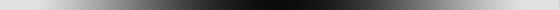Newton used to say : "If I have seen farther, it is by standing on the shoulders of giants". Because Lorentz was standing on Newton's shoulders, he saw even farther. But he still is misunderstood after 100 years. Today, we are standing on Lorentz's shoulders and we also can see farther. My contribution is a mobile spherical standing wave system which proves to match the electron behavior and also a complete and consistent wave theory about matter and all physical phenomena. It is so simple that nobody would believe it. In my opinion, the electron alone explains the whole Universe.| 01 | 02 | 03 | 04 | 05 | 06 | 07 | 08 | 09 | 10 | 11 | 12 | 13 | 14 | 15 | 16 |

| 17 | 18 | 19 | 20 | 21 | 22 | 23 | 24 | 25 | 26 | You are here. | 28 | 29 | 30 | 31 | 32 | 33 |

 Gabriel LaFreniere Bois-des-Filion in Québec. On the Internet since September 2002. Last update December 3, 2009.